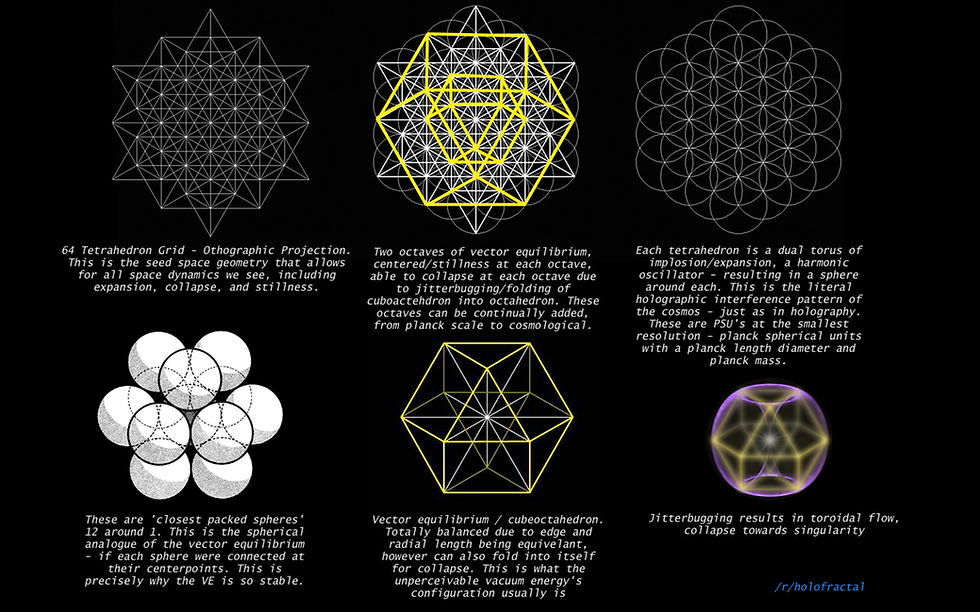top of page
Search

# superstring theory tree of life part 28

Updated: Apr 11, 2021strings(from string theory) are waves(frequency) that are harmonic numbers(mean) that come from the (fruit of life(metatrons cube(64 tetrahedron grid(E8(xen particle))))) meaning the shape of strings is 64 tetrahedron gridSome interesting arithmetic related to the number 432:

• 4+3+2=9

• 9×9=81

• 1/81 = 12345678

• 8/81= 98765432

• 98765432 x 9 = 888888888

• 12345678 x 9 = 111111111

• 432×432=186624 (close to the accepted universal constant speed of light)

• 186624 /9 /9 /9 /9 /9 = 3.16 (similar to the value of pi)

• In the so-called “magic number” sequence of the periodic table of elements – 2,8,20,28,50,82,126 = sum = 316 (see immediately above).

• 12 x 360 = 4320 (link between the number 12 and 432, degrees in a circle, etc.)

• The numbers one to 9, arranged in sequence in groups of three:

• 1 2 3 4 5 6 7 8 9

• The middle vertical, horizontal, and both diagonal rows add to 15. The perimeters add to 6, 12, 18, and 24 respectively.